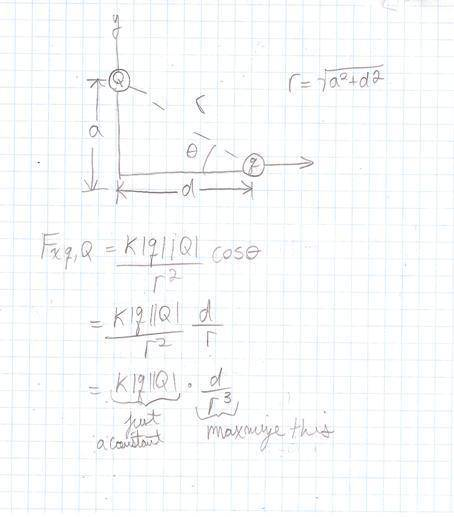# Maximum x component force on a particle given orientation

jegues

## Homework Statement

A particle with charge Q is located on the Y axis a distance a from the origin. A second particle with a charge q is played on the X axis a distance d from the origin. The value of d for which the x component of the force on the second particle is the greatest is:

a)0, b)a, c)root(2)*a, d) a/2, e) a/root(2)

## The Attempt at a Solution

Here's my attempt at the problem so far,I determined the x component of the force on the 2nd particle due to the first and I see what portion of the equation I must maximize but I don't see how to actually choose d (or solve d) such that it is maximized in terms of a.

Any ideas?

## Answers and Replies

Homework Helper
Gold Member
I determined the x component of the force on the 2nd particle due to the first and I see what portion of the equation I must maximize but I don't see how to actually choose d (or solve d) such that it is maximized in terms of a.

Any ideas?
So far, so good!I can see from your figure that you already know that $$r = \sqrt{a^2 + d^2}$$.

Plug that into your d/r3, then maximize the result with respect to the variable d (treat everything else as a constant [even a]).jegues
So far, so good!I can see from your figure that you already know that $$r = \sqrt{a^2 + d^2}$$.

Plug that into your d/r3, then maximize the result with respect to the variable d (treat everything else as a constant [even a]).Yes I knew this portion, but what I don't know how to maximize it. I should have made my question clearer.

Can you clarify?

Homework Helper
Gold Member
Yes I knew this portion, but what I don't know how to maximize it. I should have made my question clearer.

Can you clarify?
To maximize a function, take the derivative with respect to whatever variable you wish to vary, and then set the result equal to zero. Solve for the variable (algebra). That's the value of the variable that maximizes or minimizes the function.*

*The answer can give local maxima or local minima. So if you get multiple values (such as in a quadratic), you might wish to do some sanity checking to figure out what each value is. In this particular problem though, you end up with a single maximum (no minimum) so the need for sanity checking doesn't apply here.

The idea behind the procedure is this: When a smooth function rises to the top of its [possibly local] maximum or bottom of its [possibly local] minimum, the slope of the line is zero at this point. So the above procedure is merely finding where the slope of the function equals zero, as the variable changes.

jegues
To maximize a function, take the derivative with respect to whatever variable you wish to vary, and then set the result equal to zero. Solve for the variable (algebra). That's the value of the variable that maximizes or minimizes the function.*

*The answer can give local maxima or local minima. So if you get multiple values (such as in a quadratic), you might wish to do some sanity checking to figure out what each value is. In this particular problem though, you end up with a single maximum (no minimum) so the need for sanity checking doesn't apply here.

The idea behind the procedure is this: When a smooth function rises to the top of its [possibly local] maximum or bottom of its [possibly local] minimum, the slope of the line is zero at this point. So the above procedure is merely finding where the slope of the function equals zero, as the variable changes.

At the start the algebra looked a little ugly but then I remembered since we are setting this expression equal to 0 I only needed to consider the numerator.

I was able to conclude that the correct answer is,

$$d = \frac{a}{\sqrt{2}}$$

Thanks again!